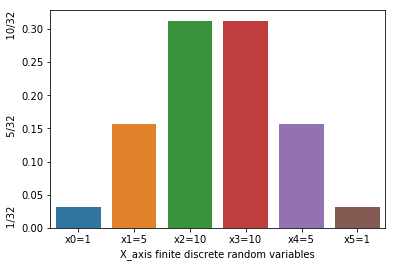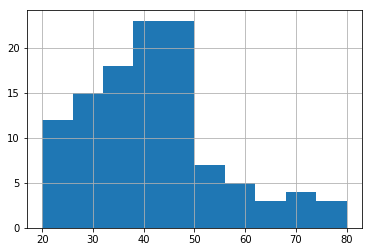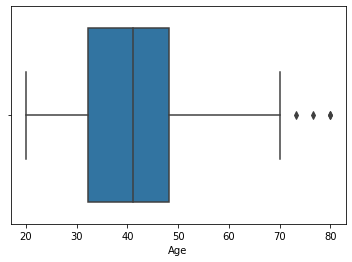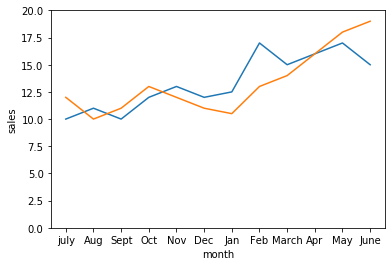Introduction to Data Visualization

Bar Chart: A bar chart or bar graph is a chart or graph that presents categorical data with rectangular bars with heights or lengths proportional to the values that they represent. The bars can be plotted vertically or horizontally. A vertical bar chart is sometimes called a line graph.

A bar graph is a nice way to display categorical data.

Below is the pictorial representation for the bar chart

[codesyntax lang=”python”]

```import numpy as np
import pandas as pd
import matplotlib.pyplot as plt
import seaborn as sns```

[/codesyntax]

[codesyntax lang=”python”]

```prob={"X_axis finite discrete random variables":['x0=1','x1=5','x2=10','x3=10','x4=5','x5=1'],'col2':[1/32,5/32,10/32,10/32,5/32,1/32]}
prob=pd.DataFrame(prob)
a=sns.barplot(x='X_axis finite discrete random variables',y='col2',data=prob).set_ylabel('1/32                      5/32                        10/32')```Histogram: A histogram is an accurate representation of the distribution of numerical data. It differs from a bar graph, in the sense that a bar graph relates two variables, but a histogram relates only one. To construct a histogram, the first step is to “bin” (or “bucket”) the range of values—that is, divide the entire range of values into a series of intervals—and then count how many values fall into each interval. The bins are usually specified as consecutive, non-overlapping intervals of a variable. The bins (intervals) must be adjacent, and are often (but are not required to be) of equal size.

A histogram is a best way to display continuous data.

Below is the pictorial representation for the histogram:

[codesyntax lang=”python”]

```generated_ages_of_people=(np.linspace(20,30,21).tolist()+np.linspace(30,40,31).tolist()
+np.linspace(40,50,40).tolist()
+np.linspace(50,60,10).tolist()
+np.linspace(60,70,6).tolist()
+np.linspace(70,80,4).tolist()
+np.linspace(80,90,1).tolist())
data_frame=pd.DataFrame(generated_ages_of_people,columns=['Age'])
ax=data_frame.Age.hist()
ax.set_xlabel("AGE")
ax.set_ylabel("Number of people")```

[/codesyntax]Box whisker plot: A box and whisker plot—also called a box plot—displays the five-number summary of a set of data. The five-number summary is the minimum, first quartile, median, third quartile, and maximum.

In a box plot, we draw a box from the first quartile to the third quartile. A vertical line goes through the box at the median. The whiskers go from each quartile to the minimum or maximum.

Box plots are non-parametric: they display variation in samples of a statistical population without making any assumptions of the underlying statistical distribution.

Here is the example of the box whisker plot:

[codesyntax lang=”python”]

`sns.boxplot(x="Age",data=data_frame)`

[/codesyntax]Line plot: This shows the trend of the data. The scale is very important when comparing two or more line plots.

A line chart allows us to track the development of several variables at the same time. It is best to use a line plot when comparing fewer than 25 numbers.

[codesyntax lang=”python”]

```monthly_sales={'month':['july','Aug','Sept','Oct','Nov','Dec','Jan','Feb','March','Apr','May','June'],\
'price':[10,11,10,12,13,12,12.5,17,15,16,17,15], \
'sales':[12,10,11,13,12,11,10.5,13,14,16,18,19]}
sales_df=pd.DataFrame(monthly_sales)

plt=sns.lineplot(x='month',y='price',data=sales_df,sort=False,sizes=[1,20]).set(ylim=(0, 20))
plt=sns.lineplot(x='month',y='sales',data=sales_df,sort=False,sizes=[1,20]).set(ylim=(0, 20))```

[/codesyntax]Scatter plot: A scatter plot is a two-dimensional data visualization that uses dots to represent the values obtained for two different variables – one plotted along the x-axis and the other plotted along the y-axis.

Below is the example for the scatter plot:

[codesyntax lang=”python”]

```rng = np.random.RandomState(0)
x = rng.randn(100)
y = rng.randn(100)
colors = rng.rand(100)

plt.scatter(x, y, c=colors, alpha=0.9, cmap='viridis')```

[/codesyntax]–By

Categories:## 2 thoughts on “Introduction to Data Visualization for Data Scientist”

1.instagram.com.es says:

The first 4 options you have mentioned are all BI tools and not necessarily for visualization. You need to create the cubes and the infrastructure to get the reports out of them.

2.Youtube Proxy says:

some truly marvellous work on behalf of the owner of this website , absolutely great content material.

DataJango - Chatbot